Browse by Topic
Related Topics

# In Mathematica, Pictures Are Worth a Thousand Words

One of the challenges of developing Mathematica is resisting the urge to spend all my time playing with the graphics toys I create. A lot of what I do results in features so fun to explore that they jeopardize the further development of Mathematica. I’d like to point out a few of them in this blog, starting with a simple but profound change in the behavior of Mathematica graphics: direct graphics output.

In previous versions of Mathematica, the result of a Plot or other graphics command was the abbreviated form  - Graphics -  that represented the symbolic output. The actual graphical image itself was spit out like a watermelon seed as a side-effect of the evaluation and was not associated with the symbolic output.

In Mathematica 6, the output and the image are one and the same, behavior we call “direct output” to contrast it with the “side-effect output” of previous versions. This simple change in behavior underlies much of the interesting new functionality in Version 6.

An immediate consequence of direct output is that it is now easy to make lists of graphics: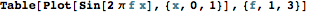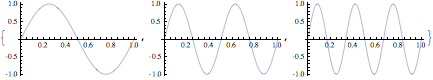(In previous versions, the output would have been:
{- Graphics -, - Graphics -, - Graphics -}”.)

Tables of graphics are straightforward, too: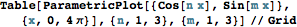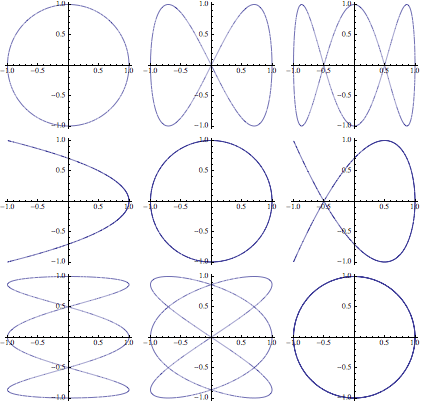In fact, graphics and text are now so tightly integrated that graphics can appear anywhere in a typeset expression: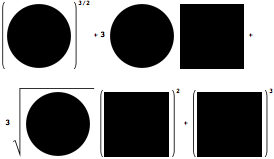That’s all fine and good, but where did the symbolic output of graphics evaluations go? Answer: the image is the symbolic output. How can that be possible?

All Mathematica expressions have both input and output forms. The input form is the text you type when you enter an expression, for example:

1/Sqrt[x]

The output form is the way the expression is presented in output—in this case, as a two-dimensional typeset fraction and radical: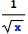As far as Mathematica is concerned, those are equivalent representations of the same expression. They can be used interchangeably.

In Mathematica 6, we made graphics follow the same paradigm. When you evaluate a graphics expression, the image you see is the output form of the corresponding Graphics or Graphics3D expression. For the first time, the image and the textual expression are equivalent, and any output can be reused as input—that’s where the fun begins.

Let’s say you want to know the options of a Plot output. Type “// Options” after the image and evaluate.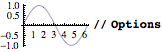{AspectRatio -> 1/GoldenRatio, Axes -> True, AxesOrigin -> {0, 0}, PlotRange -> {{0, 2 Pi}, {-1., 1.}}, PlotRangeClipping -> True, PlotRangePadding -> {Scaled[0.02], Scaled[0.02]}}

Want to add a label to your plot? Just Append the PlotLabel option to the image.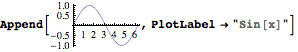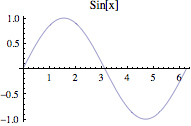Replacement operations are especially fun. Replacing Line with Polygon in this plot output gives a filled plot.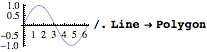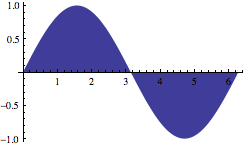Similarly, replacing Line with Point shows the vertices of the plot curve—revealing Mathematica’s adaptive plot refinement strategy, which places points more densely in the regions of a plot that have high curvature.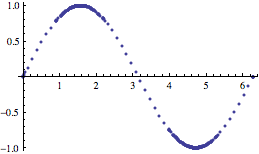Of course, 3D graphics work the same way. Here’s a ParametricPlot3D of a cone. Adding black edges to the polygons that make up the cone’s surface reveals how Mathematica subdivides the polygonal mesh to yield a smooth surface.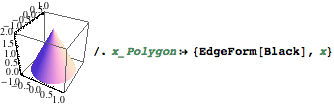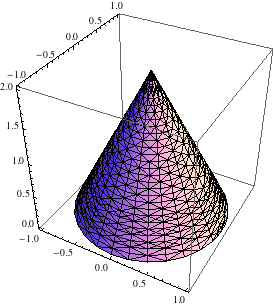Notice that all of the symbolic information necessary to describe an image lurks not far behind the image itself. That makes it possible in Mathematica 6 to define functions that grab an image’s symbolic description, rearrange or otherwise modify the description and instantly update the image. Hook that function up to a button and put the button into a notebook, and you have the beginnings of a palette that operates on graphics. It’s all incredibly easy in Mathematica 6.